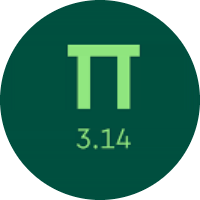# Learning the Multiplication and Division

In this quiz, You will learn about the multiplication and division concept. You will find problems on each type of multiplication and division. This quiz will surely help you in practicing the multiplication and division.

Start Quiz

Multiply.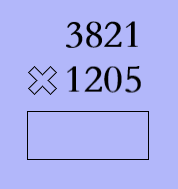47,67,325

46,04,305

67,54,985

Multiply.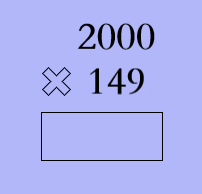2,98,000

3,88,000

3,98,000

Evaluate.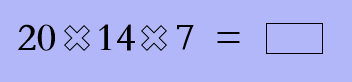2920

1960

980

Which sign makes the statement true?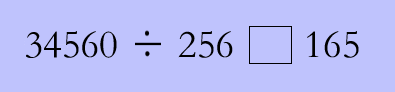>

=

<

Divide.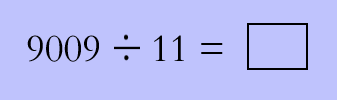349

819

569

Evaluate.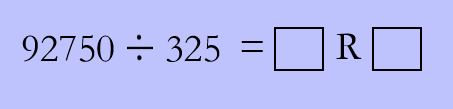285, R = 125

275, R =225

375, R = 185

A grocery store mailed 4 coupons to each person in their town. There are 512 people in the town. How many coupons did the store mail in all?

2048

2342

2400

In the gym class, each student runs 9 laps. There are 11 students in each gym class. How many laps will be run by one class during 2 gym sessions?

212

110

198

A climbing gym employee needs to order 8000 centimeters of rope. How many packages of rope should be ordered if there are 400 centimeters of rope in each?

50

40

20

Is 456,348 divisible by 5?

Yes

No

Quiz/Test Summary
Title: Learning the Multiplication and Division
Questions: 10
Contributed by: